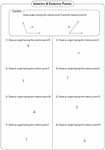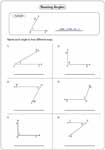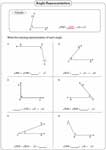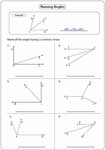1. Worksheets>
2. Math>
3. Geometry>
4. Angles>
5. Parts of Angles

Identifying Parts and Naming Angles Worksheets

This page is broadly classified into two sections, 'parts of an angle' and 'representing angles'. The first section contains identifying vertex and sides of an angle, identifying the position of points and setting up own examples. The second section contains naming angles in different ways.

Parts of an Angle

Name the Vertex and Sides

Each worksheet has 9 problems identifying vertex and sides of an angle.Your own Angle: Vertex and Sides

Draw any angle with the given vertex and sides. Students should use only the ruler to complete these worksheets. Answers may vary.Identifying Position of Points

A point can be either interior, exterior or on the angle. Tell the location of the points.Your own Angle: Interior and Exterior Points

A point is given in each problem. Follow the direction and draw the angle. Answers may vary.Representing Angles

Naming Angles

Each worksheet has six problems in naming angles. Represent each angle in four different ways.Missing Angle Representation

Three of the four possible angle representations are given. Find the missing one.Common Vertex

There are two or more rays with a common vertex. Name each angle sharing the same vertex.The entire collection of worksheets on identifying parts and naming angles can be downloaded in a jiffy!

Related Worksheets